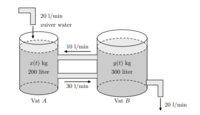# applied differential equations

#### Maxim

##### New member
Hi it is almost time for my exams, and to prepare us our professor gave us some questions he asked the previous years. I have solved almost all of them but the final one has me completely baffled. the question goes as follows:

Barrel A is filled with 200 L of salt solution. We call x(t) the amount of kg salt in barrel A (t in minuts). Barrel B is filled with 300 L salt solution and y(t) is the amount of kg of salt in barrel B. Each minute 10L flows from B to A and 30L flows from A to B. on top of that there is a tap above barrel A which adds 20l/min to barrel a and a drain underneath barrel B which removes 20l/min. Because of this the overall volume level remains the same.

a) proof that x(t) and y(t) follow the following Differential equations: - x'(t) = (-3/20)x(t) + (1/30)y(t)
- y'(t) = (3/20)x(t) - (1/10)y(t)
b) get y from the fist equation en substitute in the second equation to form a second order diff. equation in x and solve (still constants in answer)
c) If you know that there was 20kg of salt in barrel A at time 0 and 15kg in barrel b what are the formulas for x(t) and y(t) (remove the constants).

In general I find stuff to "proof that x = y" to be very difficult as I never quite know where to start. If anybody could help me out with some sort of general strategy that would be most apreciated.

there is a image of the set-up added.

-kind regards
Maxim#### HallsofIvy

##### Elite Member
Is it the word "prove" (NOT "proof") that bothers you? This problem is just asking you to set up the differential equation that describes the situation.

Barrel A is filled with 200 L of salt solution. We call x(t) the amount of kg salt in barrel A (t in minutes)."
So the concentration of salt in A is x(t)/200 kg/L.

"Barrel B is filled with 300 L salt solution and y(t) is the amount of kg of salt in barrel B."
The concentration of salt in B is y(t)/300 kg/L.

"Each minute 10L flows from B to A and 30L flows from A to B."
That 10L flowing from B to A contains 10(y/300)= y/30 kg of salt per minute and the 30L flowing from A to B contains 30(x/200)= 3x/20 kg of salt per minute. So far the rate at which the amount of salt in A is changing is dx/dt= y/30- 3x/20 kg/min and the rate at which the amount of salt in B is changing is dy/dt= 3x/20- y/30 kg/min.

"on top of that there is a tap above barrel A which adds 20l/min to barrel a and a drain underneath barrel B which removes 20l/min. Because of this the overall volume level remains the same."
I presume that tap is adding 20 L/min of pure water so doesn't change the amount of salt in A. But the tap draining B drains 20(y/300)= 2y/30 kg/min of salt. Subtracting that from what we had above, dy/dt= 3x/20- y/30- 2y/30= 3x/20- 3y/30= 3x/20- y/10.

So we have dx/dt= y/30- 3x/20 and dy/dt= 3x/20- y/10.

#### Maxim

##### New member
thank you very much for your swift reply. the problem was that I was not thinking of using concentration but instead working directly with the mass. thanks to you questions b and c where quite easy to solve after part a.

#### HallsofIvy

##### Elite Member
You were asked to find mass but the information you were given was about the flow of water. You have to make the connection between the two- and that connection is the concentration.